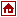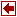## Nonlinear Programming Packages

 NLPQL Author K. Schittkowski University of Bayreuth Faculty of Mathmatics and Physics D-95440 Bayreuth Germany Language FORTRAN-77 Algorithm NLPQL solves general nonlinear mathematical programming problems with equality and inequality constraints. It is assumed that all problem functions are continuously differentiable. The internal algorithm is a sequential quadratic programming (SQP) method. Proceeding from a quadratic approximation of the Lagrangian function and a linearization of the constraints, a quadratic subproblem is formulated and solved by the dual code QL. Subsequently a line search is performed with respect to two alternative merit functions and the Hessian approximation is updated by the modified BFGS-formula. Input Format Modeling Languages link Commercial Status For more details contact the author. E-mail: klaus.schittkowski@uni-bayreuth.de Platform Any machine with a reasonable amount of memory and a Fortran compiler. Remarks NLPQL is written in double precision FORTRAN-77 and organized in form of a subroutine. Nonlinear problem functions and analytical gradients must be provided by the user within special subroutines or the calling program. Special Features: upper and lower bounds on the variables handled separately, reverse communication (evaluation of function values in main program), scaling of function values, initial multiplier and Hessian approximation, bounds and linear constraints remain satisfied, full documentation by initial comments, References K. Schittkowski, NLPQL: A Fortran subroutine for solving constrained nonlinear programming problems, Annals of Operations Research, Vol. 5, 485-500 (1985/86)